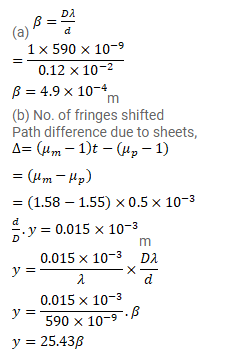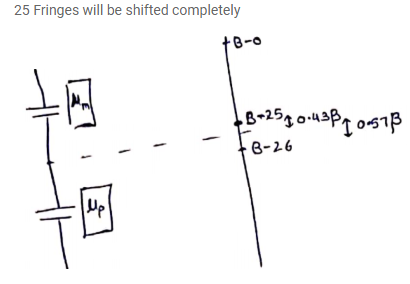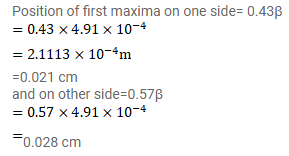# A mica strip and a polysterene strip are fitted on the two slits

Question:

A mica strip and a polysterene strip are fitted on the two slits of a double slit apparatus. The thickness of the strips is $0.50$ $\mathrm{mm}$ and the separation between the slits is $0.12 \mathrm{~cm}$. The refractive index of mica and polysterene are $1.58$ and $1.55$ respectively for the light of wavelength $590 \mathrm{~nm}$ which is used in the experiment. The interference is observed on a screen a distance one metre away. (a) What would be the fringe-width? (b) At what distance from the centre will be the first maximum be located?

Solution: